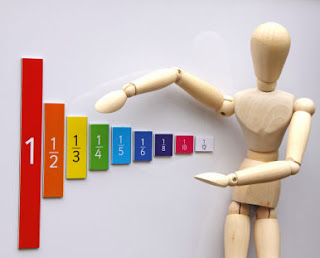## 13 January 2014

Multiplication and Division on FractionDivisions of Fractions

• To obtain the reciprocal of a fraction, interchange the numerator with denominator.
• To divide a whole number by a fraction, take the reciprocal of the fraction and then multiply it with the whole number.
• To divide a fraction by a whole number, multiply the fraction with the reciprocal of the whole number.
• To divide a fraction by a fraction, multiply the first fraction with the reciprocal of the second fraction.
Multiplication and Division on Decimals

Multiplication of Decimals

• To multiply a whole number by a decimal number, follow these steps: Ignore the decimal and multiply the two numbers.
• Count the number of digits to the right of decimal point in the original decimal number. Insert the decimal, from right to left, in the answer by the same count.
• To multiply a decimal number by a decimal number, follow these steps: Ignore the decimals and multiply the two numbers.
• Count the number of digits to the right of decimal point in both the decimal numbers.
• Add up the number of digits counted and insert the decimal, from right to left, in the answer by the same count.
To multiply a decimal number with 10, 100 or 1000, follow these steps:

• While multiplying a decimal number with 10, retain the original number and shift the decimal to the right by one place.
• While multiplying a decimal number with 100, retain the original number and shift the decimal to the right by two places.
• While multiplying a decimal number with 1000, retain the original number and shift the decimal to the right by three places.

Division of Decimals

• To divide a decimal number by a whole number, follow these steps: Convert the decimal number into a fraction.
• Take the reciprocal of the divisor. Multiply the reciprocal with the fraction.
• To divide a decimal number by another decimal number, follow these steps: Convert both the decimal numbers into fractions.
• Take the reciprocal of the divisor.
• Multiply the reciprocal with the fraction.
 With our free PDF notes you can get success in any competitive or entrance exams like CTET,  KVS, NET, CAT, MAT, CMAT, SSC, B.ED, IBPS Recruitment, IAS, CSAT, State Civil Services Exams, UPTET, PSTET, HTET & many more. It also provides NCERT solutions, CBSE, NTSE, Olympiad study material, Indian General Knowledge, English, Hindi, Mathematics, Current affairs, Science, S.ST, model test papers, important Questions and Answers asked in CBSE examinations.